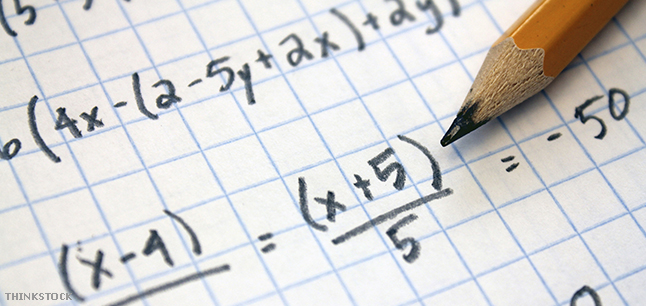# Advanced Higher Mathematics (Course Code: C847 77)

## SCQF Level 7 (32 SCQF credit points)#### Why study Mathematics?

Mathematics is important in everyday life, allowing us to make sense of the world around us and to manage our lives. Using mathematics enables us to model real-life situations and make connections and informed predictions. It equips us with the skills we need to interpret and analyse information, simplify and solve problems, assess risk and make informed decisions.

You will also get to develop skills in the areas of algebra, geometry and trigonometry as well as learn elementary calculus.

The skills you learn in this course are useful in many careers involving engineering, medicine, technology, business and the physical sciences.

#### What do I need to get in?

This is at the discretion of the school/college but you would normally be expected to have attained one of the following:

#### What will I study?

The course comprises three areas of study.

Methods in Algebra and Calculus

You will:

• develop advanced knowledge and skills in algebra and calculus that can be used in practical and abstract situations to manage information in mathematical form
• study partial fractions, standard procedures for both differential calculus and integral calculus
• explore methods for solving both first order and second order differential equations.

The importance of logical thinking and proof is emphasised throughout this unit.

Applications of Algebra and Calculus

You will:

• develop advanced knowledge and skills that involve the application of algebra and calculus to real-life and mathematical situations, including applications of geometry
• acquire skills in interpreting and analysing problem situations where these skills can be used
• explore binomial theorem, the algebra of complex numbers, properties of functions, rates of change and volumes of revolution
• study aspects of sequences and series, including summations, proved by induction.

Geometry, Proof and Systems of Equations

You will:

• develop advanced knowledge and skills that involve geometry, number and algebra, and to examine the close relationship between them
• develop skills in logical thinking
• explore matrices, vectors, solving systems of equations, the geometry of complex numbers, as well as processes of rigorous proof.

#### How will I be assessed?

Course Assessment

The course assessment consists of two components totalling 115 marks:

• Component 1 – Question paper 1 (non-calculator) (35 marks)
• Component 2 – Question paper 2 (80 marks).

Both question papers are set and externally marked by the Scottish Qualifications Authority (SQA).

The grade awarded is based on the total marks achieved across course assessment.

The course assessment is graded A-D.

#### What can I go on to next?

Further study, training or employment in: